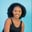Related Tags

python
python3
communitycreator

# How to use the NumPy.median() function in PythonMaria Elijah

## Overview

NumPy is a linear algebra library for Python. It allows us to work with numeric data. Numeric data can be created and stored in a data structure called a NumPy array. NumPy has various functions to perform calculations on arrays of numeric data. One of these is the median() function.

### The numpy.median() function

The function numpy. median() returns the median of a NumPy array.

### Syntax

numpy.median(
arr,
axis=None,
out=None,
overwrite_input=False,
keepdims=False
)


### Parameters

• arr: This represents the input array.
• axis: This represents the axis on which we want to calculate the median. If the axis is 0, the direction is down the rows. If it is 1, the direction is down the columns.
• out: This is an optional parameter that saves the NumPy result. The default value for the median() function is none.
• keepdims: This argument specifies the input array’s dimension. If the value is ‘True’, the decreased axes are retained in the output.

### Example

The following code shows how to use the numpy.median() function in Python:

# import numpy
import numpy as np
# create a list
my_list = [24,8,3,4,86,42,56,34,8]
# convert the list to the numpy array
np_list = np.array(my_list)
# compute the median and store it
np_list_median = np.median(np_list)

print(f"The median is {np_list_median}")
An example of the np.median() method

### Explanation

• Line 2: We import the numpy library.
• Line 4: We create a list called my_list.
• Line 6: We convert the list to a NumPy array and store it in a variable, np_list.
• Line 8: We use the np.median() and compute the median for the np_list.
• Line 10: We display the result.

RELATED TAGS

python
python3
communitycreator

CONTRIBUTORMaria Elijah
RELATED COURSES

View all Courses

Keep Exploring

Learn in-demand tech skills in half the time